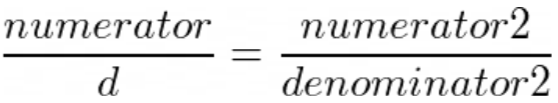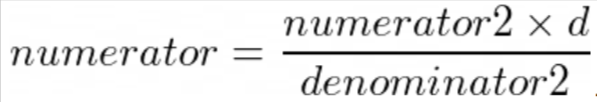AmirReza PoorAkhavana.poorakhavan

13min

# Problem of June '21

Again I’m here with a new problem to discuss. This month we hadn’t so many problems, but I selected a nice problem from TCO2B; IncorrectCancellation!

The problem had a low acceptance rate so suitable for Problem of the month. Also, it was attractive for me, personally. Also, the problem doesn’t need any special prerequisite so it makes it easy for everyone to think on and learn.

Problem statement: Given d, find a numerator such that:

• After removing some digits from d (name it diff) we get denominator2.

• After removing some digits from the numerator (name it diff2) we get numerator2.

• Multiset of diff = multiset of diff2.

• The equality satisfies.The first thing that came to my mind was that the number of d’s that have the positive answers is not so much. But it is surely wrong. At least any number divisible by 10 has a positive answer.

Hint: The number of digits are not so much, so the number of subsets of digits (to be removed) are not so much.

Fix the subset of digits that will remain after removing some digits and name it denominator2 (note that the first digit of denominator2 must be non-zero). There are 2^(number of digits of d) - 2 options.
Also, let’s name the multiset of removed digits diff.

``````1
2
3
4
5
6
7
8
9
10
11
12
13
14
``````d_digits = [int(x) for x in str(d)]
for mask in range(1, (1 << len(d_digits)) - 1):
denominator2 = 0
first_digit_is_zero = False
diff = ""
for i in range(len(d_digits)):
if mask >> i & 1:
denominator2 = denominator2 * 10 + d_digits[i]
if not denominator2:
first_digit_is_zero = True
else :
diff += str(d_digits[i])
diff = ''.join(sorted(diff))
if not first_digit_is_zero:
``````

Now fix numerator2. It can be any number in the range [1, denominator2). Now we can find numerator:So numerator2 * d must be divisible by denominator 2.

``````1
2
``````for numerator2 in range(1, denominator2):
if d * numerator2 % denominator2 == 0:
``````

Another condition is the multiset of digits that are removed from d to make denominator2 be equal to the multiset of digits that are removed from numerator to make numerator2.
To check this, we remove numerator2 digits from numerator and check if the multiset of remaining digits are equal to diff.

The complete code here:

``````1
2
3
4
5
6
7
8
9
10
11
12
13
14
15
16
17
18
19
20
21
22
23
24
25
26
27
28
29
30
31
``````class IncorrectCancellation:
def find(self, d):
d_digits = [int(x) for x in str(d)]
for mask in range(1, (1 << len(d_digits)) - 1):
denominator2 = 0
first_digit_is_zero = False
diff = ""
for i in range(len(d_digits)):
if mask >> i & 1:
denominator2 = denominator2 * 10 + d_digits[i]
if not denominator2:
first_digit_is_zero = True
else :
diff += str(d_digits[i])
diff = ''.join(sorted(diff))
if not first_digit_is_zero:
for numerator2 in range(1, denominator2):
if d * numerator2 % denominator2 == 0:
numerator = d * numerator2 // denominator2
numerator2_str = str(numerator2)
j = 0
diff2 = ""
for digit in str(numerator):
if j < len(numerator2_str) and digit == numerator2_str[j]:
j += 1
else :
diff2 += digit

if j == len(numerator2_str) and ''.join(sorted(diff2)) == diff:
return numerator
return -1
``````

### Problem of February '21

Again, I’m with you and here is the problem of February 2021. I selected MinMaxGame, as it’s game and games ar...